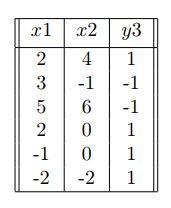# NPTEL Deep Learning Assignment 1 Answers 2023Deep Learning has received a lot of attention over the past few years and has been employed successfully by companies like Google, Microsoft, IBM, Facebook, Twitter etc. to solve a wide range of problems in Computer Vision and Natural Language Processing. In this course we will learn about the building blocks used in these Deep Learning based solutions. Specifically, we will learn about feed forward neural networks, convolutional neural networks, recurrent neural networks and attention mechanisms.

### NPTEL Deep Learning Assignment 1 Answers 2023:

1. The table below shows the temperature and humidity data for two cities. Is the data linearly separable?

Yes

No

Cannot be determined from the given information

2. What is the perceptron algorithm used for?

Clustering data points

Finding the shortest path in a graph

Classifying data

Solving optimization problems

3. What is the most common activation function used in perceptrons?

Sigmoid

ReLU

Tanh

Step

4. Which of the following Boolean functions cannot be implemented by a perceptron?

AND

OR

XOR

NOT

5. We are given 4 points in R2 say, x1=(0,1),x2=(−1,−1),x3=(2,3),x4=(4,−5).Labels of x1,x2,x3,x4 are given to be −1,1,−1,1 We initiate the perceptron algorithm with an initial weight w0=(0,0) on this data. What will be the value of w0 after the algorithm converges? (Take points in sequential order from x1 to x)( update happens when the value of weight changes) (0,0)

(−2,−2)

(−2,−3)

(1,1)

6. We are given the following data:Can you classify every label correctly by training a perceptron algorithm? (assume bias to be 0 while training)

Yes

No

7. Suppose we have a boolean function that takes 5 inputs x1,x2,x3,x4,x5? We have an MP neuron with parameter θ=1. For how many inputs will this MP neuron give output y=1

21

31

30

32

8. Which of the following best represents the meaning of term “Artificial Intelligence”?

The ability of a machine to perform tasks that normally require human intelligence

The ability of a machine to perform simple, repetitive tasks

The ability of a machine to follow a set of pre-defined rules

The ability of a machine to communicate with other machines

9. Which of the following statements is true about error surfaces in deep learning?

They are always convex functions.

They can have multiple local minima.

They are never continuous.

They are always linear functions.